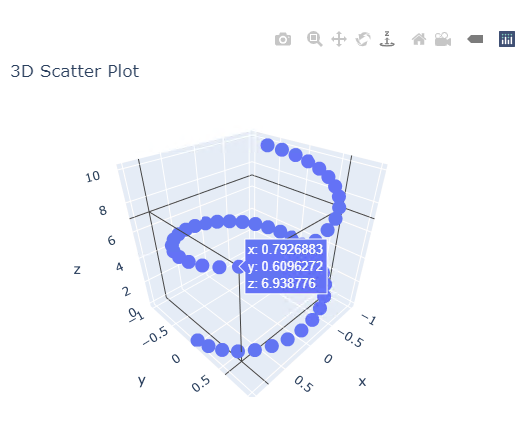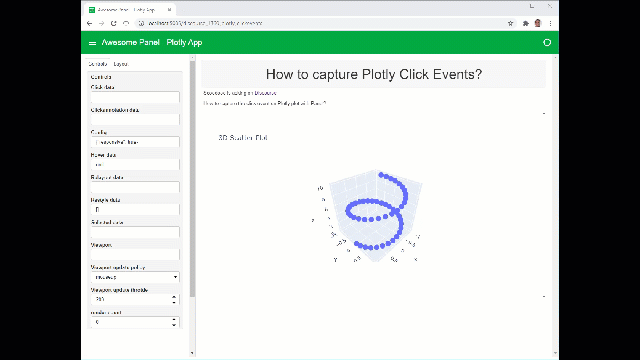# How to capture the click event on Plotly plot with Panel?

Below is a simple 3D scatter plot generated with Panel + Plotly in JupyterLab.
Does anyone how to capture the clicked coordinate (x,y,z) within the Plotly chart as shown below? I need to retrieve this (x,y,z) to drive a different plot. Thanks.

``````import numpy as np
import plotly.graph_objs as go
import panel as pn
pn.extension('plotly')

t = np.linspace(0, 10, 50)
x, y, z = np.cos(t), np.sin(t), t
fig = go.Figure(
data=go.Scatter3d(x=x, y=y, z=z, mode='markers'),
layout=dict(title='3D Scatter Plot')
)

pn.pane.Plotly(fig)
``````Hi @scoodood.

It’s a good question. You can use the `click_data` parameter on the Plotly pane.

It’s not directly documented in the Reference Guide. Feel free to create a FR or PR.!

Inspired by the last example in the Plotly reference guide and your code I created the below. It was just so fun and easy with the new templates.plotly_click|video

``````"""

How to capture the click event on Plotly plot with Panel?
"""

import numpy as np
from panel.template.react import ReactTemplate
import plotly.graph_objs as go
import panel as pn

pn.extension("plotly")
pn.config.sizing_mode = "stretch_width"

def create_plot():
t = np.linspace(0, 10, 50)
x, y, z = np.cos(t), np.sin(t), t
fig = go.Figure(
data=go.Scatter3d(x=x, y=y, z=z, mode="markers"), layout=dict(title="3D Scatter Plot")
)
fig.layout.autosize = True
return fig

def create_layout(plot):
description_panel = pn.layout.Card(
__doc__, header="# How to capture Plotly Click Events?", sizing_mode="stretch_both"
)
plot_panel = pn.pane.Plotly(plot, config={"responsive": True}, sizing_mode="stretch_both")

template = ReactTemplate(title="Awesome Panel - Plotly App")
template.sidebar.append(settings_panel)
template.main[0, :] = description_panel
template.main[1:4, :] = plot_panel
return template

def create_app():
plot = create_plot()
return create_layout(plot)

app = create_app()
app.servable()
``````
1 Like

I did a little tweet about it. Feel free to share if you like.

2 Likes

hi @Marc, thanks for the sample code. I am totally new to `Panel`. I have been trying to study your code and learn how to use param. But I am still not sure how to access the `click_data`. Can you please show me to how print “hello world” when the `click_data` is triggered? Thanks.

Sure. Here you go

``````import numpy as np
import panel as pn
import plotly.graph_objs as go

pn.extension("plotly")
pn.config.sizing_mode = "stretch_width"

def create_plot():
t = np.linspace(0, 10, 50)
x, y, z = np.cos(t), np.sin(t), t
fig = go.Figure(
data=go.Scatter3d(x=x, y=y, z=z, mode="markers"), layout=dict(title="3D Scatter Plot")
)
fig.layout.autosize = True
return fig

plot = create_plot()
plot_panel = pn.pane.Plotly(plot, config={"responsive": True}, sizing_mode="stretch_both")

@pn.depends(plot_panel.param.click_data, watch=True)
def print_hello_world(click_data):
print("hello world", click_data)

@pn.depends(plot_panel.param.click_data)
def string_hello_world(click_data):
return click_data

app= pn.Column(plot_panel, string_hello_world)

app.servable()
``````
1 Like

hi @Marc, wow this is a lot simpler than I thought. Thank you very much.

1 Like

Thanks for asking for a simple example. And thanks for your positive feedback.